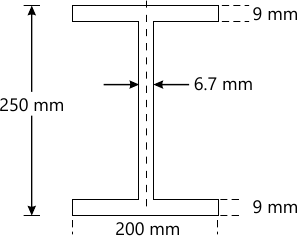MORE IN Elements of Civil Engg. & Engg. Mechanics
VTU First Year Engineering (P Cycle) (Semester 1)
Elements of Civil Engg. & Engg. Mechanics
December 2013
Total marks: --
Total time: --
INSTRUCTIONS
(1) Assume appropriate data and state your reasons
(2) Marks are given to the right of every question
(3) Draw neat diagrams wherever necessary

Choose the correct answer for the following :-
1 (a) (i) A bridge constructed at some angle to river flow is
(A) skew bridge
(B) square bridge
(C) steel bridge
(D) lift bridge
1 M
1 (a) (ii) The structure that separates roads into separate lanes is called
(A) Kerb
(B) Median
(D) camber
1 M
1 (a) (iii) The upstream side of a dam
(A) arch dams
(B) gravity dam
(C) earth dam
(D) reservoir
1 M
1 (a) (iv) Geotechnical engineering involves the study of
(A) Water
(B) Soil
(C) Air
(d) None of these
1 M
1 (b) With the help of neat sketches, briefly explain the cross-section of road and gravity dam.
10 M
1 (c) What are infrastructure related projects?
6 M

Choose the correct answer for the following :-
2 (a) (i) The component of a force perpendicular to its line of action is
(A) Maximum
(B) Minimum
(C) Zero
(D) None of the
1 M
2 (a) (ii) The moment of a force about a moment centre lying on its line of action is
(A) Maximum
(B) Minimum
(C) Zero
1 M
2 (a) (iii) Two equal and opposite forces separated by a distance will produce
(A) Translation
(B) rotation
(C) both translation and rotation
(D) none of these
1 M
2 (a) (iv) Moment of a force will produce
(A) translation
(B) rotation
(C) both translation and rotation
(D) none of these
1 M
2 (b) A block weighing w=10 kN is resting on an inclined plane as shown in Fig.Q2(b). Determine its component normal to and parallel to the inclined plane. The plane makes an angle of 20° with the horizontal.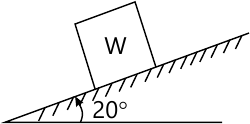4 M
2 (c) A body is subjected to the three forces as shown in Fig.Q2(c). If posssible determine the direction of the force "F" so that resultant is in 'x' direction when (i) F=5000 N, (ii) F=300 N.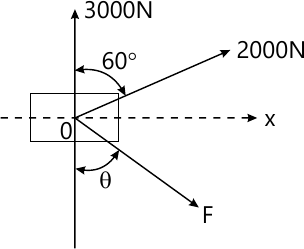12 M

Choose the correct answer for the following :-
3 (a) (i) In case of coplanar concurrent force the resultant force passes through
(A) Point of concurrece
(B) Away from point of concurrence
(C) different plane
(D) none of these
1 M
3 (a) (ii) If two concurrent forces each of 'P' act at right angles to each other, their resultant is
(A) 2P
(B) P
(C) ?2 P
(D) 2?P
1 M
3 (a) (iii) If &sume;V=0 and ?H=0 for a coplanar noncocurrent force system, then it is in
(A) equilibrium
(B) translation
(C) rotation
(D) none of these
1 M
3 (a) (iv) Conditions of equilibrium for a coplanar concurrent force system is
(A) one
(B) two
(C) three
(D) four
1 M
3 (b) Two forces acting on a body are 500 N and 1000 N as shown in fig. Q3(b). Determine the third forces "F" such that the resultant of all the three forces is 1000 N directed at 45° to 'x' axis.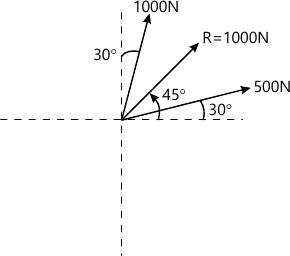6 M
3 (c) Determine the resultant of the four forces acting on a body as shown in Fig.Q3(c) with respect to point "O".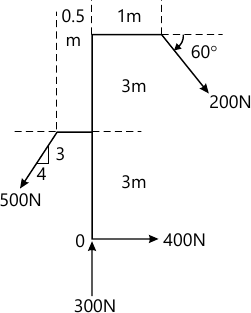10 M

Choose the correct answer for the following :-
4 (a) (i) The centroid of a triangle of height 'h' is located at a _____ distance from its apex.
(A) h/2
(B) 2h/3
(C) h/3
(D) h
1 M
4 (a) (ii) Intersection of ______ number of symmetrical axes will give centroid of plane area.
(A) 3
(B) 4
(C) 2
(D) none of these
1 M
4 (a) (iii) Moment of an area about a reference axis passing through its centroid is
(A) maximum
(B) minimize
(C) zero
(D) none of these
1 M
4 (a) (iv) Centroid of a semicircle from an axis passing through the diameter is
$(A)\ \dfrac {4r}{3\pi}\\(B)\ \dfrac {3r}{4\pi}\\(C)\ \dfrac {3\pi}{4r}\\(D)\ \dfrac {4\pi}{3r}\\$
1 M
4 (b) Determine the centroid of a semi-circular lamina of radius "r" by the method of intergration.
6 M
4 (c) Determine the centroid of the shaded area shown in the Fig. Q4(c) with respect to OX and OY.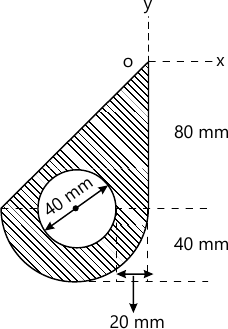10 M

Choose the correct answer for the following :-
5 (a) (i) If three forces are acting at a point and are in equilibrium, out of which two are acting in the same line, then the third force is
(A) maximum
(B) minimum
(C) zero
(D) none of these
1 M
5 (a) (ii) A rigid body is in equilibrium if the resultant force of concurrent force system is
(A) positive
(B) negative
(C) zero
(D) none of these
1 M
5 (a) (iii) Lami's theorem is valid for ______ number of concurrent forces in equilibrium.
(A) two
(B) three
(C) four
(D) none of these
1 M
5 (a) (iv) The force equal and opposite to resultant is called as
(A) equilibriant
(B) similar force
(C) opponent force
(D) none of these
1 M
5 (b) State and prove Lami's theorem.
6 M
5 (c) The frictionless pulley 'A' shown in Fig. Q5(c) is supplied by two bars AB and AC which are hinged at 'B' and 'C' to a vertical wall. The flexible cable DG hinged at 'D', goes over the pulley and supports a load of 20 kN at 'G'. The angles between the various members are shown in the figure. Determine the force in the bars AB and AC. Neglect the size and weight of the pulley.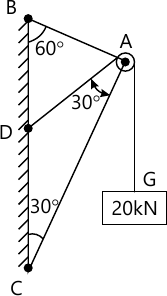10 M

Choose the correct answer for the following :-
6 (a) (i) A hinged support can have _____ reactions.
(A) 2
(B) 4
(C) 1
(D) none of these
1 M
6 (a) (ii) A determine beam can have ______ number of unknowns.
(A) 2
(B) 3
(C) 1
(D) 4
1 M
6 (a) (iii) A fixed support can have _____ reactions.
(A) 1
(B) 2
(C) 3
(D) 4
1 M
6 (a) (iv) uld stands for
1 M
6 (b) The cantilever beam shown in Fig.Q6(b) is fixed at 'A' and is free at 'B'. Determine the reaction when it is loaded as shown.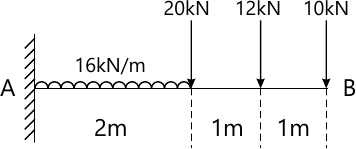6 M
6 (c) Find the force in all members of truss loaded s shown in the Fig. Q6(c). Tabulate the results.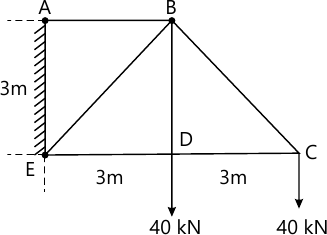10 M

Choose the correct answer for the following :-
7 (a) (i) Compared to static friction, kinetic friction is
(A) Greater
(B) Smaller
(C) Very large
(D) Zero
1 M
7 (a) (ii) Friction force is ______ to the contact surface between bodies.
(A) parallel
(B) perpendicular
(C) tangential
(D) none of these
1 M
7 (a) (iii) Friction force is ______ force.
(A) active
(B) passive
(C) normal
(D) none of these
1 M
7 (a) (iv) The tangent of the angle of friction is ______
(A) angle of repose
(B) coefficient of friction
(C) cone of friction
(D) limiting friction
1 M
7 (b) Define : -
(i) Angle of friction
(ii) Coefficient of friction
(iii) Cone of friction
6 M
7 (c) What is the value of 'P' in the system shown in Fig. Q7 (c), to cause the motion to impend to the right? Assume the pulley is smooth and coefficient of friction between the other contact surfaces is 0.20.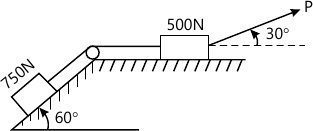10 M

Choose the correct answer for the following :-
8 (a) (i) Unit of second moment of area is
(A) m
(B) m2
(C) m4
(D) m5
1 M
8 (a) (ii) Unit of radius of gyration is ______
(A) m
(B) m2
(C) m3
(D) m4
1 M
8 (a) (iii) Moment of inertia of a square of side 'b' about an axis through its centroid is
$(A)\ \dfrac {b^4}{12}\\(B)\ \dfrac {b^4}{8}\\(C)\ \dfrac {b^4}{36}\\(D)\ \dfrac {b^3}{12}\\$
1 M
8 (a) (iv) Pdar moment of inertia is
(A) Ixx + Iyy
(B) Ixx + Izz
(C) Iyy + Izz
(D) none of these
1 M
8 (b) Define :-
(i) Moment of inertia